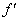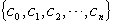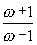### 三、代数方程的根的隔离

[傅立叶-布当判别法] f(x)=0为实系数n次代数方程，a,b为二实数，适合a<bf(a)0,f(b)0,f(x)的各阶导数为

f(x),(x),L ,f (n)(x)

{ f(b),(b),L ,f (n)(b)}

[笛卡儿符号法则]

f(x)=a0xn+a1xn-1+L +an=0 (a00,an0)

{a0,a1,L ,an}

[斯图姆判别法] f(x)为区间(a,b)内的无重根的实系数多项式，a,b为二实数，适合a<b,f(a)0,f(b)0,f0(x)表示f(x)，以f1(x)表示f(x)的导数(x).f1(x)f(x)，并以f2(x)表示由这个除法所得到的余式反号后的多项式，然后用f2(x)f1(x)，并以f3(x)表示余式反号后的多项式，

(*序列的变号次数定义如下：设两个相邻数都不为零，它们的符号相反，则称两数之间有一次变号，否则变号次数为零.如果遇到零时则应考虑该数后面第一个非零数是否变号.也就是说把序列中的一切零去掉再考虑变号次数. )

{f0(x),f1(x),f2(x),L ,fs(x)} (1)

{f0(a),f1(a),f2(a),L ,fs(a)}

{f0(b),f1(b),f2(b),L ,fs(b)}

[卢斯判别法] 假设实系数多项式

f(z)=zn+a1zn1+L +an1z+an

f0(t)=t na2tn2+a4tn4-a6tn6+L

f1(t)=a1tn1a3tn3+a5tn5L

{f0(t),f1(t),f2(t),L ,fs(t)} (2)

f(z)=0在虚轴及右半平面上没有根的充分必要条件是：斯图姆组（2）内s=n，且每个多项式的次数比前一个低一次，首项系数都是正数.

f(z)=0在右半平面上没有根而在虚轴上有p个根的充分必要条件是：斯图姆组（2）内s=n-p，且每个多项式的次数比前一个低一次，首项系数都是正数，且最后的p次方程

fnp(z)=0p个实根.这些实根就是f(z)=0在虚轴上的p个根的虚部.

z=[胡尔威茨判别法] 实系数多项式

f(z)=zn+a1zn1+L +an

f0(t)=tna2tn2+a4tn4+L

f1(t)=a1tn1a3tn3+a5tn5L